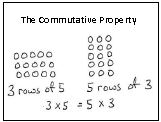3rd Module 1--Properties of Multiplication and Division

New or Recently Introduced Terms

Commutative Property (e.g., rotate a rectangular array 90 degrees to demonstrate that factors in a multiplication sentence can switch places)Multiplication/Mutiply

3 X 5 = 15
Factors Product

Divide/Divison (e.g., 15 ÷ 5 = 3)

15÷5 = 3
Factors Quotient

Unknown (e.g., with reference to a factor, missing factor)

3 X __ = 15

Unknown

15 ÷ __ = 3